# Rotate a matrix by 90 degree without using any extra space | Set 2

• Difficulty Level : Medium
• Last Updated : 24 Nov, 2021

Given a square matrix, turn it by 90 degrees in anti-clockwise direction without using any extra space.

Examples:

Attention reader! Don’t stop learning now. Get hold of all the important DSA concepts with the DSA Self Paced Course at a student-friendly price and become industry ready.  To complete your preparation from learning a language to DS Algo and many more,  please refer Complete Interview Preparation Course.

In case you wish to attend live classes with experts, please refer DSA Live Classes for Working Professionals and Competitive Programming Live for Students.

```Input:
1  2  3
4  5  6
7  8  9
Output:
3  6  9
2  5  8
1  4  7
Rotated the input matrix by
90 degrees in anti-clockwise direction.

Input:
1  2  3  4
5  6  7  8
9 10 11 12
13 14 15 16
Output:
4  8 12 16
3  7 11 15
2  6 10 14
1  5  9 13
Rotated the input matrix by
90 degrees in anti-clockwise direction.```

An approach that requires extra space is already discussed in a different article:
Inplace rotate square matrix by 90 degrees | Set 1

This post discusses the same problem with a different approach which is space-optimized.
Approach: The idea is to find the transpose of the matrix and then reverse the columns of the transposed matrix.
Here is an example to show how this works.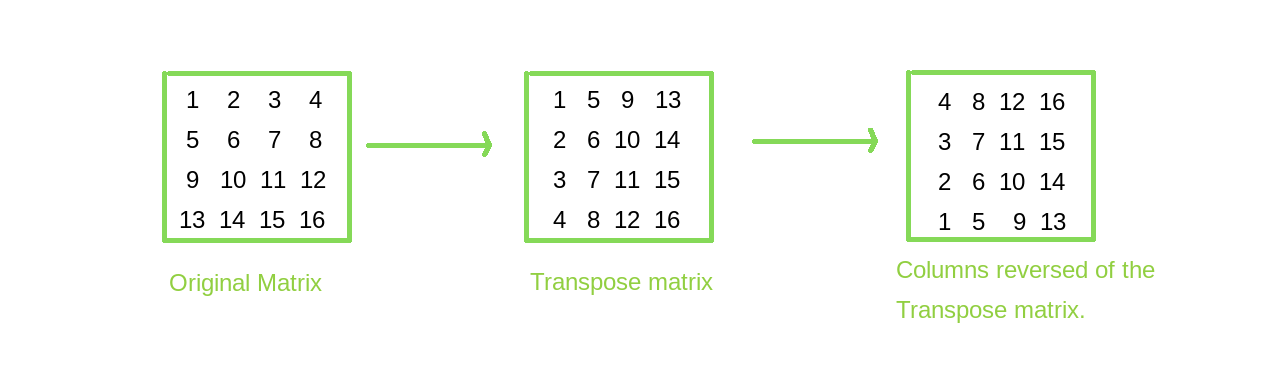Algorithm:

1. To solve the given problem there are two tasks. 1st is finding the transpose and second is reversing the columns without using extra space
2. A transpose of a matrix is when the matrix is flipped over its diagonal, i.e the row index of an element becomes the column index and vice versa. So to find the transpose interchange the elements at position (i, j) with (j, i). Run two loops, the outer loop from 0 to row count and inner loop from 0 to index of the outer loop.
3. To reverse the column of the transposed matrix, run two nested loops, the outer loop from 0 to column count and inner loop from 0 to row count/2, interchange elements at (i, j) with (i, row[count-1-j]), where i and j are indices of inner and outer loop respectively.

## C++

 `// C++ program for left``// rotation of matrix by 90 degree``// without using extra space``#include ``using` `namespace` `std;``#define R 4``#define C 4` `// After transpose we swap``// elements of column``// one by one for finding left``// rotation of matrix``// by 90 degree``void` `reverseColumns(``int` `arr[R][C])``{``    ``for` `(``int` `i = 0; i < C; i++)``        ``for` `(``int` `j = 0, k = C - 1; j < k; j++, k--)``            ``swap(arr[j][i], arr[k][i]);``}` `// Function for do transpose of matrix``void` `transpose(``int` `arr[R][C])``{``    ``for` `(``int` `i = 0; i < R; i++)``        ``for` `(``int` `j = i; j < C; j++)``            ``swap(arr[i][j], arr[j][i]);``}` `// Function for print matrix``void` `printMatrix(``int` `arr[R][C])``{``    ``for` `(``int` `i = 0; i < R; i++) {``        ``for` `(``int` `j = 0; j < C; j++)``            ``cout << arr[i][j] << ``" "``;``        ``cout << ``'\n'``;``    ``}``}` `// Function to anticlockwise``// rotate matrix by 90 degree``void` `rotate90(``int` `arr[R][C])``{``    ``transpose(arr);``    ``reverseColumns(arr);``}` `// Driven code``int` `main()``{``    ``int` `arr[R][C] = { { 1, 2, 3, 4 },``                      ``{ 5, 6, 7, 8 },``                      ``{ 9, 10, 11, 12 },``                      ``{ 13, 14, 15, 16 } };``    ``rotate90(arr);``    ``printMatrix(arr);``    ``return` `0;``}`

## Java

 `// JAVA Code for left Rotation of a``// matrix by 90 degree without using``// any extra space``import` `java.util.*;` `class` `GFG {` `    ``// After transpose we swap elements of``    ``// column one by one for finding left``    ``// rotation of matrix by 90 degree``    ``static` `void` `reverseColumns(``int` `arr[][])``    ``{``        ``for` `(``int` `i = ``0``; i < arr[``0``].length; i++)``            ``for` `(``int` `j = ``0``, k = arr[``0``].length - ``1``; j < k;``                 ``j++, k--) {``                ``int` `temp = arr[j][i];``                ``arr[j][i] = arr[k][i];``                ``arr[k][i] = temp;``            ``}``    ``}` `    ``// Function for do transpose of matrix``    ``static` `void` `transpose(``int` `arr[][])``    ``{``        ``for` `(``int` `i = ``0``; i < arr.length; i++)``            ``for` `(``int` `j = i; j < arr[``0``].length; j++) {``                ``int` `temp = arr[j][i];``                ``arr[j][i] = arr[i][j];``                ``arr[i][j] = temp;``            ``}``    ``}` `    ``// Function for print matrix``    ``static` `void` `printMatrix(``int` `arr[][])``    ``{``        ``for` `(``int` `i = ``0``; i < arr.length; i++) {``            ``for` `(``int` `j = ``0``; j < arr[``0``].length; j++)``                ``System.out.print(arr[i][j] + ``" "``);``            ``System.out.println(``""``);``        ``}``    ``}` `    ``// Function to anticlockwise rotate``    ``// matrix by 90 degree``    ``static` `void` `rotate90(``int` `arr[][])``    ``{``        ``transpose(arr);``        ``reverseColumns(arr);``    ``}` `    ``/* Driver program to test above function */``    ``public` `static` `void` `main(String[] args)``    ``{``        ``int` `arr[][] = { { ``1``, ``2``, ``3``, ``4` `},``                        ``{ ``5``, ``6``, ``7``, ``8` `},``                        ``{ ``9``, ``10``, ``11``, ``12` `},``                        ``{ ``13``, ``14``, ``15``, ``16` `} };` `        ``rotate90(arr);``        ``printMatrix(arr);``    ``}``}` `// This code is contributed by Arnav Kr. Mandal.`

## C#

 `// C# program for left rotation``// of matrix by 90 degree``// without using extra space``using` `System;` `class` `GFG {``    ``static` `int` `R = 4;``    ``static` `int` `C = 4;` `    ``// After transpose we swap``    ``// elements of column one``    ``// by one for finding left``    ``// rotation of matrix by``    ``// 90 degree``    ``static` `void` `reverseColumns(``int``[, ] arr)``    ``{``        ``for` `(``int` `i = 0; i < C; i++)``            ``for` `(``int` `j = 0, k = C - 1; j < k; j++, k--) {``                ``int` `temp = arr[j, i];``                ``arr[j, i] = arr[k, i];``                ``arr[k, i] = temp;``            ``}``    ``}` `    ``// Function for do``    ``// transpose of matrix``    ``static` `void` `transpose(``int``[, ] arr)``    ``{``        ``for` `(``int` `i = 0; i < R; i++)``            ``for` `(``int` `j = i; j < C; j++) {``                ``int` `temp = arr[j, i];``                ``arr[j, i] = arr[i, j];``                ``arr[i, j] = temp;``            ``}``    ``}` `    ``// Function for print matrix``    ``static` `void` `printMatrix(``int``[, ] arr)``    ``{` `        ``for` `(``int` `i = 0; i < R; i++) {``            ``for` `(``int` `j = 0; j < C; j++)``                ``Console.Write(arr[i, j] + ``" "``);``            ``Console.WriteLine(``""``);``        ``}``    ``}` `    ``// Function to anticlockwise``    ``// rotate matrix by 90 degree``    ``static` `void` `rotate90(``int``[, ] arr)``    ``{``        ``transpose(arr);``        ``reverseColumns(arr);``    ``}` `    ``// Driver code``    ``static` `void` `Main()``    ``{``        ``int``[, ] arr = { { 1, 2, 3, 4 },``                        ``{ 5, 6, 7, 8 },``                        ``{ 9, 10, 11, 12 },``                        ``{ 13, 14, 15, 16 } };` `        ``rotate90(arr);``        ``printMatrix(arr);``    ``}` `    ``// This code is contributed``    ``// by Sam007``}`

## Python3

 `# Python 3 program for left rotation of matrix by 90``# degree without using extra space` `R ``=` `4``C ``=` `4` `# After transpose we swap elements of column``# one by one for finding left rotation of matrix``# by 90 degree`  `def` `reverseColumns(arr):``    ``for` `i ``in` `range``(C):``        ``j ``=` `0``        ``k ``=` `C``-``1``        ``while` `j < k:``            ``t ``=` `arr[j][i]``            ``arr[j][i] ``=` `arr[k][i]``            ``arr[k][i] ``=` `t``            ``j ``+``=` `1``            ``k ``-``=` `1` `# Function for do transpose of matrix`  `def` `transpose(arr):``    ``for` `i ``in` `range``(R):``        ``for` `j ``in` `range``(i, C):``            ``t ``=` `arr[i][j]``            ``arr[i][j] ``=` `arr[j][i]``            ``arr[j][i] ``=` `t` `# Function for print matrix`  `def` `printMatrix(arr):``    ``for` `i ``in` `range``(R):``        ``for` `j ``in` `range``(C):``            ``print``(``str``(arr[i][j]), end``=``" "``)``        ``print``()` `# Function to anticlockwise rotate matrix``# by 90 degree`  `def` `rotate90(arr):``    ``transpose(arr)``    ``reverseColumns(arr)`  `# Driven code``arr ``=` `[[``1``, ``2``, ``3``, ``4``],``       ``[``5``, ``6``, ``7``, ``8``],``       ``[``9``, ``10``, ``11``, ``12``],``       ``[``13``, ``14``, ``15``, ``16``]``       ``]``rotate90(arr)``printMatrix(arr)`

## PHP

 ``

## Javascript

 ``

Output
```4 8 12 16
3 7 11 15
2 6 10 14
1 5 9 13 ```

Complexity Analysis:

• Time complexity :O(R*C).
The matrix is traversed twice, so the complexity is O(R*C).
• Space complexity :O(1).
The space complexity is constant as no extra space is required.

Another Approach using a single traversal of the matrix :

The idea is to traverse along the boundaries of the matrix and shift the positions of the elements in 900 anticlockwise directions in each boundary. There are such (n/2-1) boundaries in the matrix.

Algorithm:

1. Iterate over all the boundaries in the matrix. There are total (n/2-1) boundaries
2. For each boundary take the 4 corner elements and swap them such that the 4 corner elements get rotated in anticlockwise directions. Then take the next 4 elements along the edges(left, right, top, bottom) and swap them in an anticlockwise direction. Continue as long as all the elements in that particular boundary get rotated in 900 anticlockwise directions.
3. Then move on to the next inner boundary and continue the process as long the whole matrix is rotated in 900 anticlockwise direction.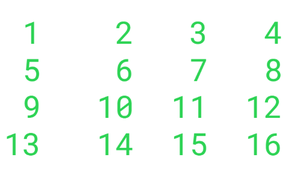Original array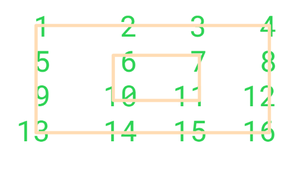total n/2-1 boundaries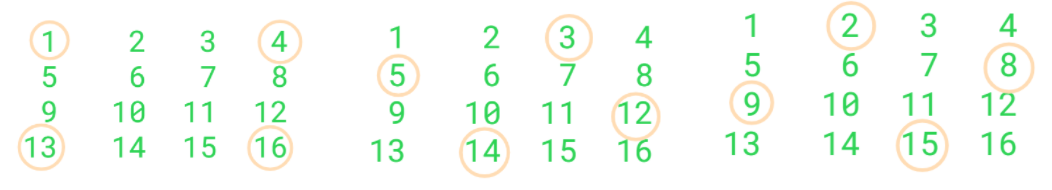for outermost boundary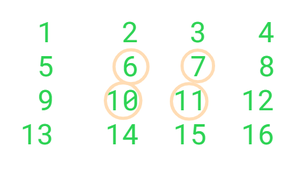for next inner boundary

## C++14

 `// C++ program for left rotation of matrix``// by 90 degree without using extra space``#include ``using` `namespace` `std;``#define R 4``#define C 4` `// Function to rotate matrix anticlockwise by 90 degrees.``void` `rotateby90(``int` `arr[R][C])``{``    ``int` `n = R; ``// n=size of the square matrix``    ``int` `a = 0, b = 0, c = 0, d = 0;` `    ``// iterate over all the boundaries of the matrix``    ``for` `(``int` `i = 0; i <= n / 2 - 1; i++) {` `        ``// for each boundary, keep on taking 4 elements (one``        ``// each along the 4 edges) and swap them in``        ``// anticlockwise manner``        ``for` `(``int` `j = 0; j <= n - 2 * i - 2; j++) {``            ``a = arr[i + j][i];``            ``b = arr[n - 1 - i][i + j];``            ``c = arr[n - 1 - i - j][n - 1 - i];``            ``d = arr[i][n - 1 - i - j];` `            ``arr[i + j][i] = d;``            ``arr[n - 1 - i][i + j] = a;``            ``arr[n - 1 - i - j][n - 1 - i] = b;``            ``arr[i][n - 1 - i - j] = c;``        ``}``    ``}``}` `// Function for print matrix``void` `printMatrix(``int` `arr[R][C])``{``    ``for` `(``int` `i = 0; i < R; i++) {``        ``for` `(``int` `j = 0; j < C; j++)``            ``cout << arr[i][j] << ``" "``;``        ``cout << ``'\n'``;``    ``}``}` `// Driven code``int` `main()``{``    ``int` `arr[R][C] = { { 1, 2, 3, 4 },``                      ``{ 5, 6, 7, 8 },``                      ``{ 9, 10, 11, 12 },``                      ``{ 13, 14, 15, 16 } };``    ``rotateby90(arr);``    ``printMatrix(arr);``    ``return` `0;``}` `// This code is contributed by Md. Enjamum``// Hossain(enja_2001)`

## Java

 `// JAVA Code for left Rotation of a matrix``// by 90 degree without using any extra space``import` `java.util.*;` `class` `GFG {` `    ``// Function to rotate matrix anticlockwise by 90``    ``// degrees.``    ``static` `void` `rotateby90(``int` `arr[][])``    ``{``        ``int` `n = arr.length;``        ``int` `a = ``0``, b = ``0``, c = ``0``, d = ``0``;` `        ``// iterate over all the boundaries of the matrix``        ``for` `(``int` `i = ``0``; i <= n / ``2` `- ``1``; i++) {` `            ``// for each boundary, keep on taking 4 elements``            ``// (one each along the 4 edges) and swap them in``            ``// anticlockwise manner``            ``for` `(``int` `j = ``0``; j <= n - ``2` `* i - ``2``; j++) {``                ``a = arr[i + j][i];``                ``b = arr[n - ``1` `- i][i + j];``                ``c = arr[n - ``1` `- i - j][n - ``1` `- i];``                ``d = arr[i][n - ``1` `- i - j];` `                ``arr[i + j][i] = d;``                ``arr[n - ``1` `- i][i + j] = a;``                ``arr[n - ``1` `- i - j][n - ``1` `- i] = b;``                ``arr[i][n - ``1` `- i - j] = c;``            ``}``        ``}``    ``}``    ``// Function for print matrix``    ``static` `void` `printMatrix(``int` `arr[][])``    ``{``        ``for` `(``int` `i = ``0``; i < arr.length; i++) {``            ``for` `(``int` `j = ``0``; j < arr[``0``].length; j++)``                ``System.out.print(arr[i][j] + ``" "``);``            ``System.out.println(``""``);``        ``}``    ``}` `    ``/* Driver program to test above function */``    ``public` `static` `void` `main(String[] args)``    ``{``        ``int` `arr[][] = { { ``1``, ``2``, ``3``, ``4` `},``                        ``{ ``5``, ``6``, ``7``, ``8` `},``                        ``{ ``9``, ``10``, ``11``, ``12` `},``                        ``{ ``13``, ``14``, ``15``, ``16` `} };` `        ``rotateby90(arr);``        ``printMatrix(arr);``    ``}``}` `// This code is contributed by Md. Enjamum``// Hossain(enja_2001)`

## C#

 `// C# Code for left Rotation of a matrix``// by 90 degree without using any extra space``using` `System;``using` `System.Collections.Generic;` `public` `class` `GFG {` `    ``// Function to rotate matrix anticlockwise by 90``    ``// degrees.``    ``static` `void` `rotateby90(``int` `[,]arr)``    ``{``        ``int` `n = arr.GetLength(0);``        ``int` `a = 0, b = 0, c = 0, d = 0;` `        ``// iterate over all the boundaries of the matrix``        ``for` `(``int` `i = 0; i <= n / 2 - 1; i++) {` `            ``// for each boundary, keep on taking 4 elements``            ``// (one each along the 4 edges) and swap them in``            ``// anticlockwise manner``            ``for` `(``int` `j = 0; j <= n - 2 * i - 2; j++) {``                ``a = arr[i + j,i];``                ``b = arr[n - 1 - i,i + j];``                ``c = arr[n - 1 - i - j,n - 1 - i];``                ``d = arr[i,n - 1 - i - j];` `                ``arr[i + j,i] = d;``                ``arr[n - 1 - i,i + j] = a;``                ``arr[n - 1 - i - j,n - 1 - i] = b;``                ``arr[i,n - 1 - i - j] = c;``            ``}``        ``}``    ``}``    ``// Function for print matrix``    ``static` `void` `printMatrix(``int` `[,]arr)``    ``{``        ``for` `(``int` `i = 0; i < arr.GetLength(0); i++) {``            ``for` `(``int` `j = 0; j < arr.GetLength(1); j++)``                ``Console.Write(arr[i,j] + ``" "``);``            ``Console.WriteLine(``""``);``        ``}``    ``}` `    ``/* Driver program to test above function */``    ``public` `static` `void` `Main(String[] args)``    ``{``        ``int` `[,]arr = { { 1, 2, 3, 4 },``                        ``{ 5, 6, 7, 8 },``                        ``{ 9, 10, 11, 12 },``                        ``{ 13, 14, 15, 16 } };` `        ``rotateby90(arr);``        ``printMatrix(arr);``    ``}``}` `// This code is contributed by umadevi9616`

## Javascript

 ``
Output

```4 8 12 16
3 7 11 15
2 6 10 14
1 5 9 13 ```

Complexity Analysis:

Time complexity :O(R*C). The matrix is traversed only once, so the time complexity is O(R*C).

Space complexity :O(1). The space complexity is O(1) as no extra space is required.

Implementation: Let’s see a method of Python numpy that can be used to arrive at the particular solution.

## Python3

 `# Alternative implementation using numpy``import` `numpy` `# Driven code``arr ``=` `[[``1``, ``2``, ``3``, ``4``],``       ``[``5``, ``6``, ``7``, ``8``],``       ``[``9``, ``10``, ``11``, ``12``],``       ``[``13``, ``14``, ``15``, ``16``]``       ``]` `# Define flip algorithm (Note numpy.flip is a builtin f``# function for versions > v.1.12.0)`  `def` `flip(m, axis):``    ``if` `not` `hasattr``(m, ``'ndim'``):``        ``m ``=` `asarray(m)``    ``indexer ``=` `[``slice``(``None``)] ``*` `m.ndim``    ``try``:``        ``indexer[axis] ``=` `slice``(``None``, ``None``, ``-``1``)``    ``except` `IndexError:``        ``raise` `ValueError(``"axis =% i is invalid for the % i-dimensional input array"``                         ``%` `(axis, m.ndim))``    ``return` `m[``tuple``(indexer)]`  `# Transpose the matrix``trans ``=` `numpy.transpose(arr)` `# Flip the matrix anti-clockwise (1 for clockwise)``flipmat ``=` `flip(trans, ``0``)` `print``(``"\nnumpy implementation\n"``)``print``(flipmat)`

Output:

```numpy implementation

[[ 4  8 12 16]
[ 3  7 11 15]
[ 2  6 10 14]
[ 1  5  9 13]]```

Note: The above steps/programs do left (or anticlockwise) rotation. Let’s see how to do the right rotation or clockwise rotation. The approach would be similar. Find the transpose of the matrix and then reverse the rows of the transposed matrix.
This is how it is done.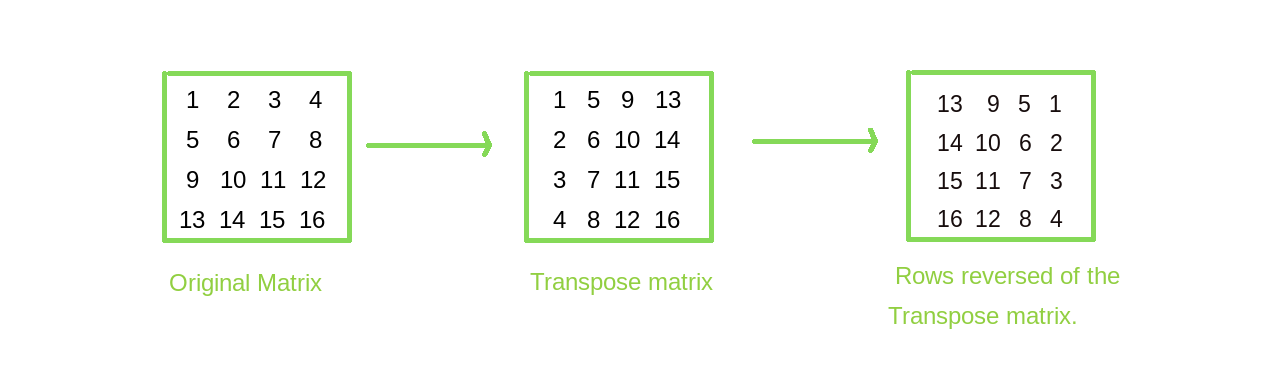This article is contributed by DANISH_RAZA . If you like GeeksforGeeks and would like to contribute, you can also write an article using write.geeksforgeeks.org or mail your article to review-team@geeksforgeeks.org. See your article appearing on the GeeksforGeeks main page and help other Geeks.

Rotating Along the Boundaries

We can start at the first 4 corners of the given matrix and then keep incrementing the row and column indices to moves around.

At any given moment we will have four corners lu (left-up),ld(left-down),ru(right-up),rd(right-down).

To left rotate we will first swap the ru and ld,  then lu and ld and lastly ru and rd.

## C++

 `#include ``using` `namespace` `std;` ` ``//Function to rotate matrix anticlockwise by 90 degrees.``void` `rotateby90(vector >& matrix)``{``      ``int` `n=matrix.size();``    ``int` `mid;``     ``if``(n%2==0)``         ``mid=n/2-1;``     ``else``         ``mid=n/2;``     ``for``(``int` `i=0,j=n-1;i<=mid;i++,j--)``     ``{``         ``for``(``int` `k=0;k>& arr)``{` `  ``int` `n=arr.size();``  ``for``(``int` `i=0;i> arr = { { 1, 2, 3, 4 },``                      ``{ 5, 6, 7, 8 },``                      ``{ 9, 10, 11, 12 },``                      ``{ 13, 14, 15, 16 } };``    ``rotateby90(arr);``    ``printMatrix(arr);``    ``return` `0;``}`

## Java

 `import` `java.util.*;` `class` `GFG{``    ``private` `static` `int``[][] swap(``int``[][] matrix, ``int` `x1,``int` `x2,``int` `y1, ``int` `y2) {``          ``int` `temp = matrix[x1][x2] ;``          ``matrix[x1][x2] = matrix[y1][y2];``          ``matrix[y1][y2] = temp;``          ``return` `matrix;``        ``}` ` ``//Function to rotate matrix anticlockwise by 90 degrees.``static` `int``[][] rotateby90(``int``[][] matrix)``{``      ``int` `n=matrix.length;``    ``int` `mid;``     ``if``(n % ``2` `== ``0``)``         ``mid = n / ``2` `- ``1``;``     ``else``         ``mid = n / ``2``;``     ``for``(``int` `i = ``0``, j = n - ``1``; i <= mid; i++, j--)``     ``{``         ``for``(``int` `k = ``0``; k < j - i; k++)``         ``{``             ``matrix= swap(matrix, i, j - k, j, i + k);     ``//ru  ld``             ``matrix= swap(matrix, i + k, i, j, i + k);     ``//lu ld``             ``matrix= swap(matrix, i, j - k, j - k, j);     ``//ru rd``          ``}``     ``}``     ``return` `matrix;``}` `static` `void` `printMatrix(``int``[][] arr)``{` `  ``int` `n = arr.length;``  ``for``(``int` `i = ``0``; i < n; i++)``  ``{``      ``for``(``int` `j = ``0``; j < n; j++)``    ``{``        ``System.out.print(arr[i][j] + ``" "``);``    ``}``    ``System.out.println();``  ``}``}``public` `static` `void` `main(String[] args) {``   ` `    ``int``[][] arr = { { ``1``, ``2``, ``3``, ``4` `},``                      ``{ ``5``, ``6``, ``7``, ``8` `},``                      ``{ ``9``, ``10``, ``11``, ``12` `},``                      ``{ ``13``, ``14``, ``15``, ``16` `} };``    ``arr = rotateby90(arr);``    ``printMatrix(arr);``}``}` `// This code is contributed by umadevi9616`

Output:

```4 8 12 16
3 7 11 15
2 6 10 14
1 5 9 13 ```

Time Complexity: O(n^2)

Space Complexity : O(1)

My Personal Notes arrow_drop_up